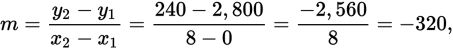# SAT Math Multiple Choice Question 501: Answer and Explanation

### Test Information

Question: 501

6. A landscaper buys a new commercial-grade lawn mower that costs \$2,800. Based on past experience, he expects it to last about 8 years, and then he can sell it for scrap metal with a salvage value of about \$240. Assuming the value of the lawn mower depreciates at a constant rate, which equation could be used to find its approximate value after x years, given that x < 8?

• A. y = -8x + 2,560
• B. y = -240x + 2,800
• C. y = -320x + 2,800
• D. y = 240x - 2,560

Explanation:

C

Difficulty: Medium

Category: Heart of Algebra / Linear Equations

Strategic Advice: Don't peek at the answers. Write your own equation using the initial cost and the rate of change in the value of the lawn mower. Remember—when something changes at a constant rate, it can be represented by a linear equation.

Getting to the Answer: When a linear equation in the form y = mx + b is used to model a real-world scenario, m represents the constant rate of change, and b represents the starting amount. Here, the starting amount is easy—it's the purchase price, \$2,800. To find the rate of change, think of the initial cost as the value at 0 years, or the point (0, 2,800), and the salvage amount as the value at 8 years, or the point (8, 240). Substitute these points into the slope formula to find thatso the equation is y = -320x + 2,800.